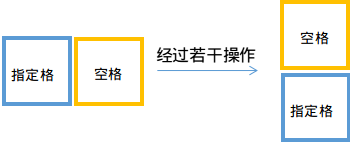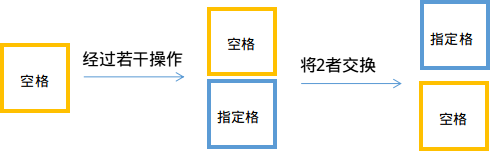# Luogu 1979 NOIP 2013 华容道（搜索，最短路径）

3 4 2
0 1 1 1
0 1 1 0
0 1 0 0
3 2 1 2 2 2
1 2 2 2 3 2

2
-1

## 解决思路1.输入数据，并预处理出$Skip[i][j][f1][f2]$
2.对于每一组询问：
(1).先让空白格移动到指定格的初始位置的周围四个格子
(2).将空白格与指定格捆绑行动，求解类最短路

## 代码

#include<iostream>
#include<cstdio>
#include<cstdlib>
#include<cstring>
#include<algorithm>
#include<queue>
using namespace std;

#define mem(Arr,x) memset(Arr,x,sizeof(Arr))

const int maxMat=40;
const int maxF=5;
const int meminf=2139062143;//这个值与memset(Arr,127,sizeof(Arr))中Arr的每一个数的值一致
const int ff={0,2,1,4,3};//ff[i]表示i的相反方向

class Pos//位置
{
public:
int x,y;
Pos()
{
return;
}
Pos(int a,int b)
{
x=a;
y=b;
}
};

class Queue_Data
{
public:
int x,y,f;
};

int n,m;
int Mat[maxMat][maxMat];
Pos F[maxF];
int Skip[maxMat][maxMat][maxF][maxF];
int Dist[maxMat][maxMat][maxF];//求解最短距离
bool vis[maxMat][maxMat][maxF];
queue<Queue_Data> Q;

Pos operator + (Pos A,Pos B);
Queue_Data operator + (Queue_Data A,Pos B);
void init();
int Bfs(Pos st,Pos ed);//从位置st走到位置ed的步数

int main()
{
int qus;//询问个数
scanf("%d%d%d",&n,&m,&qus);
for (int i=1;i<=n;i++)
for (int j=1;j<=m;j++)
scanf("%d",&Mat[i][j]);
init();//初始化Skip
while (qus--)//处理询问
{
int Epx,Epy,Stx,Sty,Glx,Gly;
scanf("%d%d%d%d%d%d",&Epx,&Epy,&Stx,&Sty,&Glx,&Gly);
if ((Mat[Stx][Sty]==0)||(Mat[Glx][Gly]==0))//当初始或目标位置本身不可走时，直接输出-1
{
printf("-1\n");
continue;
}
if ((Stx==Glx)&&(Sty==Gly))//当初始与目标位置相同时，直接输出0
{
printf("0\n");
continue;
}

while (!Q.empty())//初始化
Q.pop();
mem(Dist,127);
mem(vis,0);

//求出将空白格移动到指定格初始位置四周的步数，并将其中可行的放入队列
Mat[Stx][Sty]=0;//因为要求出空白格移动的步数，所以这时初始位置不能动，先置为0表示不可走
Pos init=(Pos){Stx,Sty};
for (int i=1;i<=4;i++)
{
Pos v=init+F[i];//枚举初始位置的四周
Dist[Stx][Sty][i]=Bfs((Pos){Epx,Epy},v);//Bfs求解从空格走到的距离
if (Dist[Stx][Sty][i]!=meminf)
Q.push((Queue_Data){Stx,Sty,i});//如果可行，放入队列
}
Mat[Stx][Sty]=1;//处理完后重新置为1

//求解最短路
while (!Q.empty())
{
Queue_Data u=Q.front();
Q.pop();
vis[u.x][u.y][u.f]=0;
for (int i=1;i<=4;i++)//枚举四个方向
{
Queue_Data v=u+F[i];
v.f=ff[i];//注意这里最后的空格方向要变为方向i的反方向
if (Dist[v.x][v.y][v.f]>Dist[u.x][u.y][u.f]+Skip[u.x][u.y][u.f][i])
{
Dist[v.x][v.y][v.f]=Dist[u.x][u.y][u.f]+Skip[u.x][u.y][u.f][i];
if (vis[v.x][v.y][v.f]==0)
{
Q.push(v);
vis[v.x][v.y][v.f]=1;
}
}
}
}

int Ans=meminf;
for (int i=1;i<=4;i++)
Ans=min(Ans,Dist[Glx][Gly][i]);//找出最小值
if (Ans==meminf)
Ans=-1;
printf("%d\n",Ans);
}
return 0;
}

Pos operator + (Pos A,Pos B)//为了方便向四周移动，重载位置的加法
{
return (Pos){A.x+B.x,A.y+B.y};
}

Queue_Data operator + (Queue_Data A,Pos B)//同上
{
return (Queue_Data){A.x+B.x,A.y+B.y,A.f};
}

void init()
{
F=(Pos){-1,0};//这个F就是在枚举向哪个方向移动时用的
F=(Pos){1,0};
F=(Pos){0,-1};
F=(Pos){0,1};
mem(Skip,127);

for (int i=1;i<=n;i++)//四重循环枚举(i,j)，空格所在方向f1，要将(i,j)移动去的方向f2
for (int j=1;j<=m;j++)
{
if (Mat[i][j]==0)//若(i,j)本身不可走则不进行操作
continue;
Mat[i][j]=0;//因为不能在移动空格的时候使(i,j)被移动，所以先置为不能走
Pos now(i,j);
for (int f1=1;f1<=4;f1++)//枚举方向
for (int f2=1;f2<=4;f2++)
{
if (f1>f2)//空格在f1，当前格走到f2和空格在f2，当前格走到f1的步数是一样的
{
Skip[i][j][f1][f2]=Skip[i][j][f2][f1];
continue;
}
Skip[i][j][f1][f2]=Bfs(now+F[f1],now+F[f2])+1;//Bfs求解
}
Mat[i][j]=1;//置回来
}
return;
}

int Bfs(Pos st,Pos ed)//求解从st走到ed的步数
{
if ((Mat[st.x][st.y]==0)||(Mat[ed.x][ed.y]==0))//当这两个点中有任意一个不可走时，直接返回无穷大
return meminf;

//各种初始化
queue<Pos> Q;
while (!Q.empty())
Q.pop();
bool vis[maxMat][maxMat];
int Dist[maxMat][maxMat];
mem(vis,0);
mem(Dist,127);

//将st放入队列
Q.push(st);
vis[st.x][st.y]=1;
Dist[st.x][st.y]=0;

do
{
Pos u=Q.front();
Q.pop();
for (int i=1;i<=4;i++)//枚举向四个方向走
{
Pos v=u+F[i];
if ((Mat[v.x][v.y]==0)||(vis[v.x][v.y]==1))
continue;
vis[v.x][v.y]=1;
Dist[v.x][v.y]=Dist[u.x][u.y]+1;
Q.push(v);
}
}
while (!Q.empty());
return Dist[ed.x][ed.y];//返回步数
}
posted @ 2017-10-01 16:27 SYCstudio 阅读(...) 评论(...) 编辑 收藏